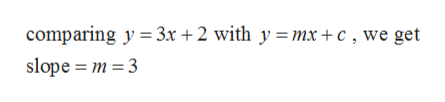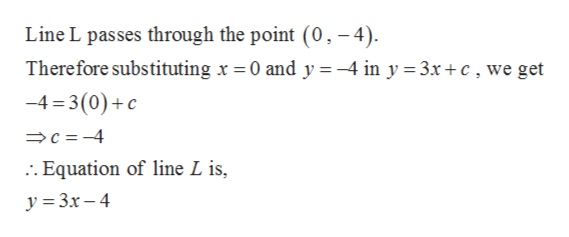# y3x+2L(8,-4)

Question
3 views

Line L in the figure below is parallel to the line y=3x+2. Find the coordinates of the point P

P=

check_circle

Step 1

First we find the slope of the given line:help_outlineImage Transcriptionclosecomparing y = 3.x + 2 with y = mx+c , we get slope m 3 fullscreen
Step 2

Since parallel lines have equal slopes, therefore slope of the line L is also 3.

Hence the equation of line L is

Step 3help_outlineImage TranscriptioncloseLine L passes through the point (0, -4) Therefore substituting x = 0 and y =-4 in y 3x+c, we get -4 3(0)c c-4 :. Equation of line L is, y 3x-4 fullscreen

### Want to see the full answer?

See Solution

#### Want to see this answer and more?

Solutions are written by subject experts who are available 24/7. Questions are typically answered within 1 hour.*

See Solution
*Response times may vary by subject and question.
Tagged in

### Other